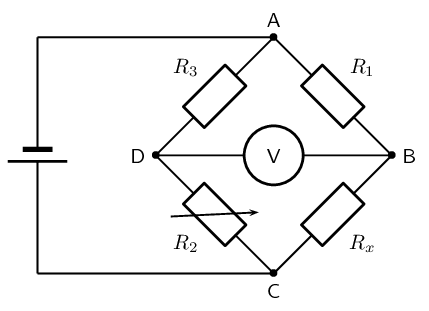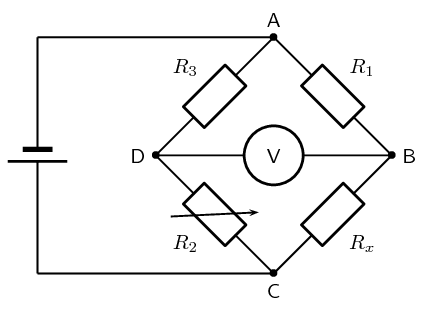Home Practice
For learners and parents For teachers and schools
Textbooks
Full catalogue
Pricing SupportLog in

We think you are located in United States. Is this correct?

# 10.5 Extension: Wheatstone bridge [Not examinable]

## 10.5 Extension: Wheatstone bridge [Not examinable] (ESCPZ)

temp text

Using what we know about parallel networks of resistors we can devise another method of finding an unknown resistance, the Wheatstone bridge. A Wheatstone bridge is a measuring instrument that is used to measure an unknown electrical resistance by balancing two legs of a bridge circuit, one leg of which includes the unknown component. Its operation is similar to the original potentiometer except that in potentiometer circuits the meter used is a sensitive galvanometer.

The Wheatstone bridge was invented by Samuel Hunter Christie in 1833 and improved and popularised by Sir Charles Wheatstone in 1843.

Despite the fact that we have given this circuit a special name, it is just a circuit containing a parallel configuration of four resistors. This is not actually a new concept, this particular configuration is just particularly useful.In the circuit of the Wheatstone bridge, $${R}_{x}$$ is the unknown resistance. $${R}_{\text{1}}$$, $${R}_{\text{2}}$$ and $${R}_{\text{3}}$$ are resistors of known resistance and the resistance of $${R}_{\text{2}}$$ is adjustable. If the ratio of $${R}_{\text{2}}$$: $${R}_{\text{1}}$$ is equal to the ratio of $${R}_{x}$$: $${R}_{\text{3}}$$, then the potential difference between the two midpoints will be zero and no current will flow between the midpoints. In order to determine the unknown resistance, $${R}_{\text{2}}$$ is varied until this condition is reached. That is when the voltmeter reads 0 V.

## Worked example 11: Wheatstone bridge

What is the resistance of the unknown resistor $${R}_{x}$$ in the diagram below if $${R}_{\text{1}} = \text{4}$$ $$\text{Ω}$$ $${R}_{\text{2}} =\text{8}$$ $$\text{Ω}$$ and $${R}_{\text{3}} =\text{6}$$ $$\text{Ω}$$.### Determine how to approach the problem

The arrangement is a Wheatstone bridge. So we use the equation:

\begin{align*} {R}_{x}:{R}_{\text{3}}& = {R}_{\text{2}}:{R}_{\text{1}} \end{align*}

### Solve the problem

\begin{align*} {R}_{x}:{R}_{\text{3}}& = {R}_{\text{2}}:{R}_{\text{1}}\\ {R}_{x}:6& = 8:4\\ {R}_{x}& = 12\phantom{\rule{3.33333pt}{0ex}}\Omega \phantom{\rule{4pt}{0ex}} \end{align*}

The resistance of the unknown resistor is $$\text{12}$$ $$\text{Ω}$$.